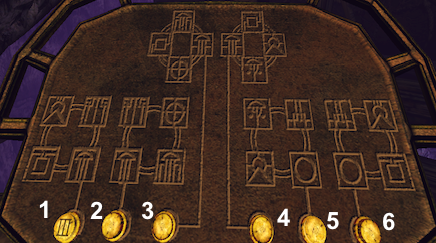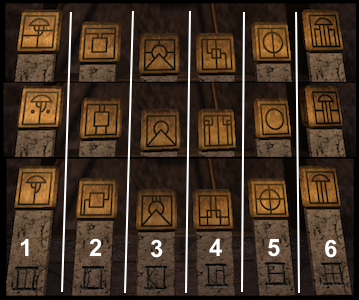# Myst VI - Uru: Ages Beyond Myst

## Kadish Tolesa - Vault Puzzle

Arguably the most difficult puzzle in Ages Beyond Myst, this puzzle requires the player to determine the unique combination of a six push-button lock. We are given the first button that must be pushed: button number one, indicated by a D'ni numeral one. We may attempt to solve this puzzle by trial and error. However there are 5 factorial, or 120 possible combinations. We will consider a much more elegant approach using mathematical logic.Figure 1. Kadish Tolesa - vault puzzle control panel.

Figure 1 shows the combination lock control panel. There are six buttons in a row. Each button is actually a toggle switch - either on or off - that must be pushed once, in the correct order, until all six buttons are pushed. Associated with each button is a set of four icons, indicated by a line drawn from the button to a group of four icons.Figure 2. Kadish Tolesa Gallery - vault puzzle icon sets.

Figure 2, shows a clue given in the Kadish Tolesa Gallery Age. The figure shows six sets of icons, each set containing three unique icons, each set shown in a column above a D'ni numeral. (In the gallery, the the icons in each set are on a rotating triangular prism.) For convenience the three icons in each set are shown in snapshots arranged in a column. At the bottom of each column is the D'ni numeral designating the cardinal number of that set.

Button #Icon Sets
Represented
above Button
Icon Sets
NOT Represented
above Button
12, 3, 4, 61, 5
24, 5, 61, 2, 3
32, 5, 61, 3, 4
41, 2, 3, 64, 5
51, 3, 4, 52, 6
62, 3, 4, 51, 6
Table 1. Icon sets represented by each control panel button.

Table 1 shows the icon sets represented by the diagram above each button in figure 1. An icon set is represented if one of its elements appears in the diagram above a button. For example, the diagram above button 1 has representatives (elements) from set numbers 2, 3, 4, and 6.

In Yeesha's journal (the one left in the Relto Age), in the chapter on the Kalish Tolesa Age, it says

Only way a man is when he is hidden is how he is. A shallow glimpse can deceive. Look deep, ponder, and recognize all that is hidden.
A somewhat obscure clue, this indicates that, in the Kalish Tolesa Age, what is hidden is what we must consider. Not immediately obvious, this clue means that we must look at the complement of the icon sets represented for each button. That is, we should look at the icon sets not represented for each button. That is why table 1 has a column to indicate, for each button, the icon sets not represented. One of these icon sets tells us the order in which that button must be pressed. Since, for each button, there are several possibilites, we must solve for the correct icon set.

To unlock the vault we must determine the order in which the buttons have to be pressed. What follows is a logical, mathematical approach to finding the correct order. First we must define a function that maps each button to a specific icon set, each indicated by a D'ni numeral in figure 2. Since each button gets pushed only once, this function must be a one-to-one function, or more precisely, a bijective function.

f : buttons → icon sets

As shown, the first button is labeled with D'ni numeral one, a clue that this button must be pressed first. Therefore we take it as a given that

f(1) = 1
We may now make the following chain of logical inferences
f(1) = 1 and f(6) ∈ {1, 6} implies f(6) = 6
f(6) = 6 and f(5) ∈ {2, 6} implies f(5) = 2
f(1) = 1 and f(5) = 2 and f(2) ∈ {1, 2, 3} implies f(2) = 3
f(1) = 1 and f(2) = 3 and f(3) ∈ {1, 3, 4} implies f(3) = 4
f(3) = 4 and f(4) ∈ {4, 5} implies f(4) = 5

A word of explanation on the above. Taking the first inference as an example:

f(1) = 1 and f(6) ∈ {1, 6} implies f(6) = 6
It must be the case that f(6) ∈ {1, 6} as these are the icon sets not represented in table 1 for button 6. That is, f(6) must be equal to either 1 or 6. Since f is, by definition, a one-to-one function, f(6) cannot equal 1, as then f(1) = f(6) = 1. Hence f would not be one-to-one, a contradiction. Therefore f(6) has to equal 6, as there are no other possible values. A similar explanation applies for each of the remaining 4 inferences.

Since the range of f represents the order in which the buttons must be pressed, the buttons must be pressed in the following order

1, 5, 2, 3, 4, 6
This opens the vault door.